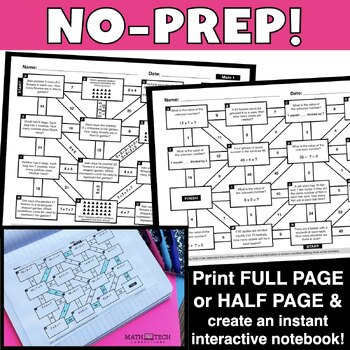DID YOU KNOW:
Seamlessly assign resources as digital activities

Learn how in 5 minutes with a tutorial resource. Try it Now

Learn More# 3rd Grade Math Mazes - 3rd Grade Math Test Prep - Fun Math Review Bundle3rd
Subjects
Standards
Resource Type
Formats Included
• Zip
Pages
25 mazes
\$20.00
Bundle
List Price:
\$25.00
You Save:
\$5.00
\$20.00
Bundle
List Price:
\$25.00
You Save:
\$5.00This bundle contains one or more resources with Google apps (e.g. docs, slides, etc.).

#### Also included in

1. Review 3rd, 4th, and 5th grade math standards with these FUN Math Mazes. This mega bundle includes 80 mazes that will keep students engaged! Use during math stations, math homework, or math test prep.Save 30% when you purchase this bundle. If you purchase individually, this bundle has a value of \$80
\$56.00
\$80.00
Save \$24.00

### Description

Review 3rd grade math standards with these FUN Math Mazes. This complete bundle includes 25 mazes that will keep students engaged! Use during math stations, math homework, or math test prep.

Save 20% when you purchase this bundle. If you purchase individually, this bundle has a value of \$25.

Currently Included:

Set 1:

Maze 1 - Multiplication (equal groups)

Maze 2 - Division (equal shares)

Maze 3 - Multiplication & Division (word problems)

Maze 4 - Multiplication & Division (unknown number in equation)

Maze 5 - Multiplication & Division (properties of multiplication)

Maze 6 - Division

Maze 7 - Division & Multiplication

Set 2:

Maze 8 - Representing Numbers

Maze 9 - Rounding (nearest 10/100)

Maze 10 - Addition within 1,000

Maze 11 - Subtraction within 1,000

Maze 12 - Addition & Subtraction within 1,000

Maze 13 - Multiply by multiples of 10

Set 3:

Maze 14 - Categorizing Shapes

Maze 15 - Partitioning Shapes

Maze 16 - Models to Represent Fractions

Maze 17 - Fractions on a Number Line

Maze 18 - Comparing Fractions

Set 4:

Maze 19 - Telling time to the nearest minute

Maze 20 - Elapsed Time

Maze 21 - Measuring Volume

Maze 22 - Measuring Mass

Maze 23 - Measuring area by counting unit squares

Maze 24 - Measuring are using multiplication

Maze 25 - Perimeter of rectangles

F.A.Q.

Are the mazes aligned to the CCSS? Yes. Not a common core state? I include a version without the standards.

Are the mazes editable? No.

Are the mazes available in digital format? Yes. I include a Google Slides version with moveable arrows.

>> More Math Mazes <<

PAPERLESS Math Resources

Total Pages
25 mazes
Included
Teaching Duration
N/A
Report this Resource to TpT
Reported resources will be reviewed by our team. Report this resource to let us know if this resource violates TpT’s content guidelines.

### Standards

to see state-specific standards (only available in the US).
Partition shapes into parts with equal areas. Express the area of each part as a unit fraction of the whole. For example, partition a shape into 4 parts with equal area, and describe the area of each part as 1/4 of the area of the shape.
Understand that shapes in different categories (e.g., rhombuses, rectangles, and others) may share attributes (e.g., having four sides), and that the shared attributes can define a larger category (e.g., quadrilaterals). Recognize rhombuses, rectangles, and squares as examples of quadrilaterals, and draw examples of quadrilaterals that do not belong to any of these subcategories.
Compare two fractions with the same numerator or the same denominator by reasoning about their size. Recognize that comparisons are valid only when the two fractions refer to the same whole. Record the results of comparisons with the symbols >, =, or <, and justify the conclusions, e.g., by using a visual fraction model.
Express whole numbers as fractions, and recognize fractions that are equivalent to whole numbers. Examples: Express 3 in the form 3 = 3/1; recognize that 6/1 = 6; locate 4/4 and 1 at the same point of a number line diagram.
Recognize and generate simple equivalent fractions, (e.g., 1/2 = 2/4, 4/6 = 2/3). Explain why the fractions are equivalent, e.g., by using a visual fraction model.